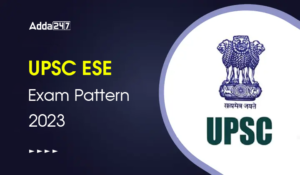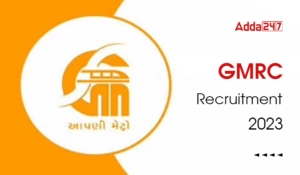Engineering Jobs   »   Civil Engineering quizs   »   UPSSSC JE QUIZ

# UPSSSC-JE’21 CE: Daily Practice Quiz. 13-November-2021

Know your strengths and practice your concepts with this quiz on UPSSSC-JE Recruitment 2021. This quiz for UPSSSC-JE Recruitment 2021 is designed specially according to UPSSSC-JE Syllabus 2021.

Quiz: Civil Engineering
Exam: UPSSSC-JE
Topic: MISCELLANEOUS

Each question carries 1 mark
Negative marking: 1/4 mark
Time: 8 Minutes

Q1. It was note that on a section of road, the free speed was 70 kmph and the jam density was 80 vpkm. The maximum flow in vph that could be expected on this road is
(a) 800
(b) 1400
(c) 2800
(d) 5600

Q2. The absolute minimum radius of curve for safe operation, for a speed of 100 kmph is:
(a) 260 m
(b) 440 m
(c) 360 m
(d) 320 m

Q3. A rectangular open channel of width 5.0 m is carrying a discharge of 100m³/s. The Froude number of the flow is 0.8. The depth of flow (in m) in the channel is
(a) 4
(b) 5
(c) 16
(d) 20

Q4. Two reservoirs are connected by two pipes laid in parallel. The diameters and lengths of the two pipe is same, but the friction factor for the first pipe is 4 times than the second pipe; The ratio of the discharges in first pipe to the second pipe will be
(a) 4
(b) 2
(c) 0.5
(d) 0.25

Q5. What is the approximate length of convergent cone of a venturimeter? ‘D’- diameter of inlet section and ‘d’- diameter of throat.
(a) 2 (D-d)
(b) 2.5 (D-d)
(c) 2.7 (D-d)
(d) 2.25 (D-d)

Q6. A pitot tube is used to measure the velocity of water in a pipe. The stagnation pressure head is 6 m and static pressure head is 5m. Calculate the velocity of flow assuming the coefficient of tube equal to 0.98
(a) 2.34 m/sec
(b) 3.34 m/sec
(c) 4.34 m/sec
(d) 5.34 m/sec

SOLUTION

S1. Ans.(b)
Sol. Maximum flow = (free speed × Jam density)/4
=(70×80)/4
= 1400 veh/hour.

S2. Ans.(c)
Sol. Given,
Minimum design speed V_min = 100 kmph
e_max = 0.07, f = 0.15 (Normally)
R_Absolute=V^2/127(e+f)
=(100)^2/127(0.07+0.15)
= 357.9 m ≃ 360 m

S3. Ans. (a)
Sol.Q = 100 m³/sec.
Froude No. (fr) = 0.8
Depth of flow (y) = ?

Fr = v/√gy = Q/(A√gy) = 100/(5×y√(g×y))

0.8 = 100/(5 × y √(g×y) )
0.8 × 5 × y × √(9.81×y) = 100
y = 3.99m ≈ 4.0 m

S4. Ans.(c)
Sol. Pipe (1) Pipe (2)
Diameter = d Diameter = d
Length = L Length = L
Friction factor = 4f Friction factor = f
→ If two pipe connected Parallel than head loss at both is equal
hf₁ = hf₂
(8Q_1^2)/π²g (F₁L₁)/〖D_1〗^5 = (8Q₂²)/π²g (f_2 L₂)/(D₂^5 )
(Q_1^2×4f×L)/d^5 = (Q_2^2×f×L)/d^5
(Q_1^2)/(Q_2^2 ) = 1/4
(Q₁)/(Q₂) = 1/2=0.5

S5. Ans. (b)
Sol. Length of Convergent Cone of venturimeter

2.5 (D – d)

Length of Divergent cone of venturimeter
7.5 (D – d)

S6. Ans. (c)
Sol. V = Cv√2gh
h = (Pstagnation )/ρg – (Pstatic )/ρg
h = 6 – 5

h = 1 m

V = 0.98 × √(2×9.81×1)

V = 4.34 m/sec

Sharing is caring!

Thank You, Your details have been submitted we will get back to you.
•GATE 2023 Admit Card Out, Direct Link fo...
•UPSC ESE Admit Card 2023, Download Preli...
•BHEL Engineer Trainee Syllabus and Exam ...
•UPSC ESE Exam Pattern 2023, Check UPSC E...
•GMRC Recruitment 2023, Apply Online For ...
•UPSC ESE Previous Year Cutoff, Check Yea...
•UPSC ESE/IES 2023 Notification, Apply On...